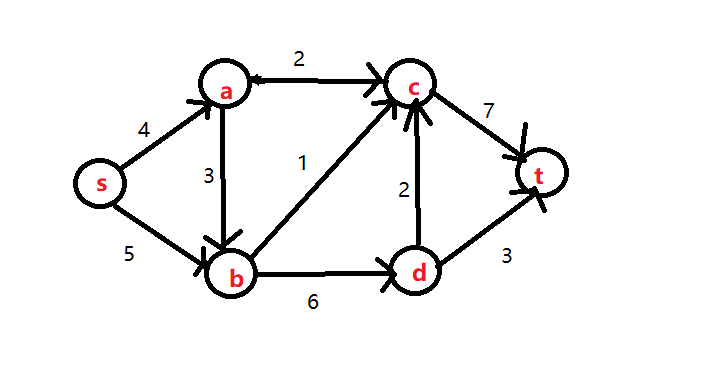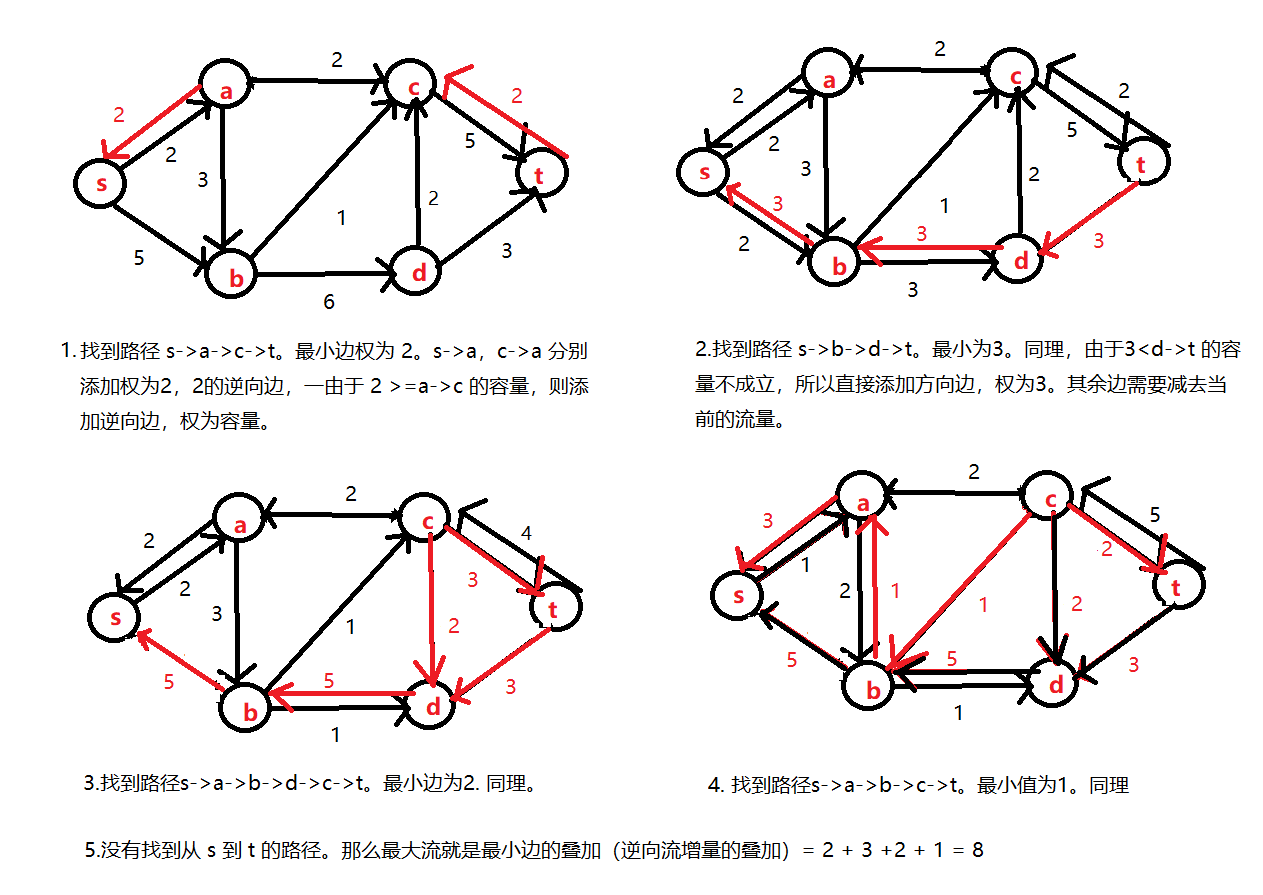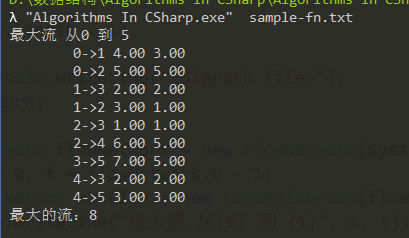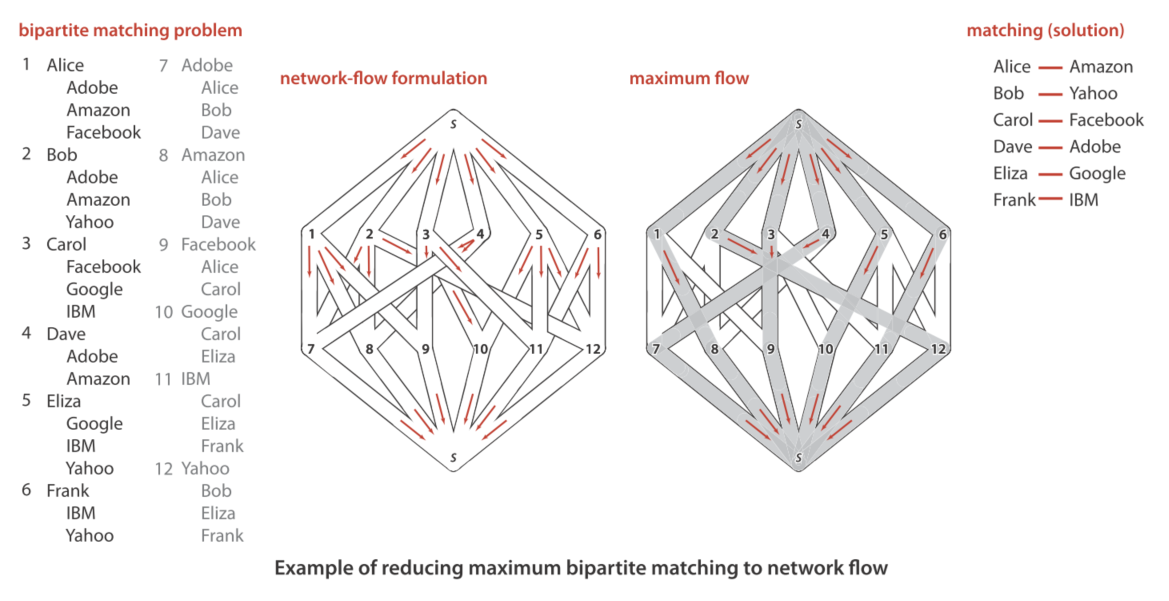# 网络流

• 容量限制：f(u, v) <= c(u, v) 一条边的流不超过它的容量
• 反对称性：f(u,v) = -f(v,u)。
• 流量守恒：除非 u=s 或 u=t，否则从 u 流入流出的总和必须是 0。

f(u,v) 为从 u 发送到顶点 v 的流量（flow）。流的值定义为 |f|=从源点 s 到 t 的最大流。

• 经过边的流量不能超过流量
• 除了源点 s 和汇点 t，对于其他的点，满足输入流量=输出流量## Ford-Fulkerson 算法

• 残留网路（Residual networks）
• 增广路径（Augmenting paths）
• 割（Cut）

### 最大流-最小割定理

1. 存在某个 st- 切分，其容量和 f 的流量相等
2. f 达到了最大流量
3. f 中已经不存在任何的增广路径

Ford-Fulkerson 算法是一种迭代的方法。有以下的步骤：

1. 设当前的流为 0， 也就是对于 u,v 有 f(u, v) = 0
2. 在当前的图中找到一条从 s 到 t 的路径。如果没有，则表示不存在增广路径了（没有更小的流量可以作为逆向边的增量），停止，最大流即是增量和
3. 计算路径上所有的有向边的最小值，记为 bottle
4. 对所有的路径中的每条有向边 <u,v> 更新当前的剩余流量 = 容量- bottle
5. 让增量和累加上 bottle

### 剩余网络## 二分图匹配

• 每个顶点都有输入和输出，总是会有一个合法的匹配
• 每条边的权为 1，也就是每个顶点最多出现在一个匹配上。
• 匹配不可能包含有更多的边，因为还有更多类似的匹配。# 线性规划（待续）

• 0 <= x(s->a) <= 4 1
• 0 <= x(s->b) <= 5 2
• 0 <= x(a->b) <= 3 3
• 0 <= x(a->c) <= 2 4
• 0 <= x(b->c) <= 1 5
• 0 <= x(b->d) <= 6 6
• 0 <= x(c->t) <= 7 7
• 0 <= x(d->c) <= 2 8
• 0 <= x(d->t) <= 3 9
• 守恒：x(s->a) = x(a->b) + x(a->c)
• 守恒：x(s->b) + x(a->b) = x(b->d) + x(b->c)
• 守恒：x(a->c) + x(b->c) + x(d->c) = x(c->t)
• 守恒：x(b->d) = x(d->c) + x(d->t)

# 参考

1. （Cnblogs）Ford-Fulkerson 最大流算法
2. （Wikipedia）最大流最小割定理
3. （Wikipedia）Ford-Fulkerson 算法
4. （Wikipedia）网络流
5. Robert Sedgewick等，算法（第四版）[M]. 北京：人民邮电出版社，2012：580-597
6. Frank R. Giordano 等，数学建模（第五版）[M]. 北京：机械工业出版社，2014：235-243
7. （Renfei's blog）二分图的最大匹配